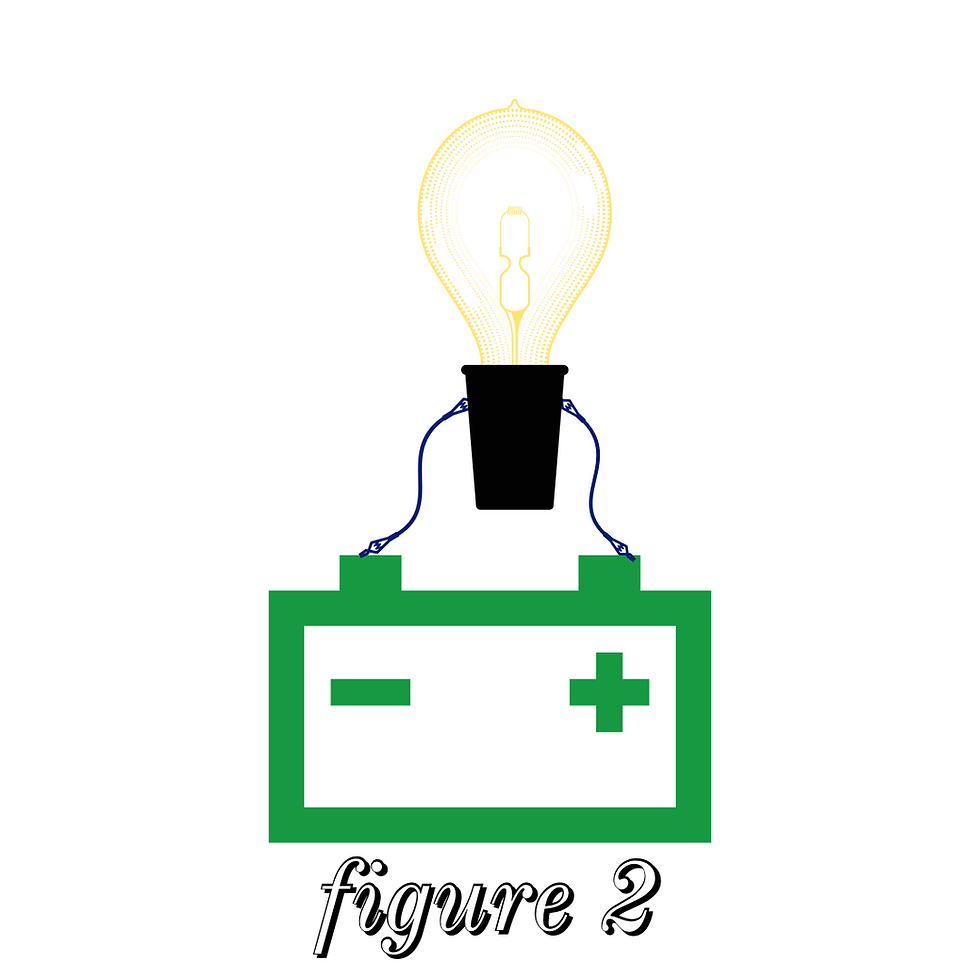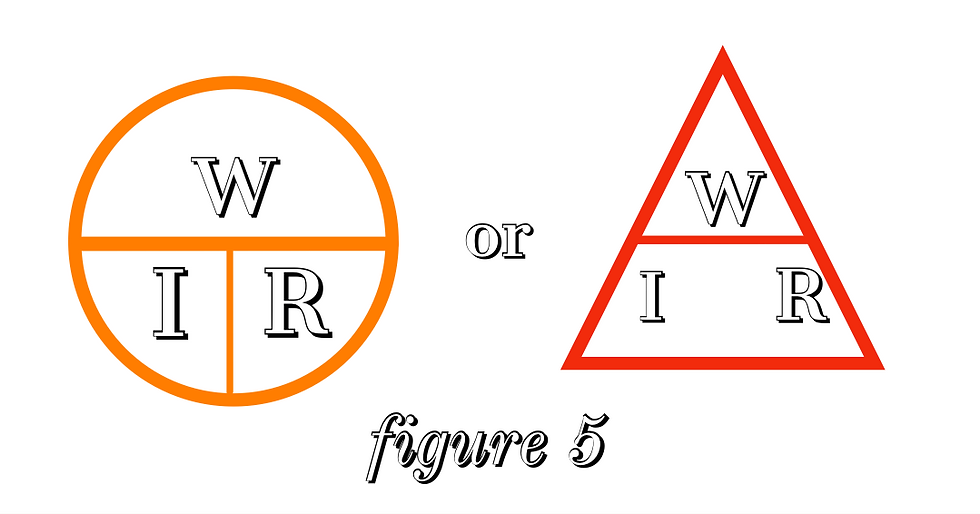top of page
Search
• Leonard J. Williams

# Electricity and Magnets - from the Magna-Lock USA archives

Updated: Jul 23, 2021

Electricity is one the greatest forces known to man. Its number of uses is unlimited. Scientists have (by studying electricity) found that all things--liquids, solids, and gases--are made up of very small electrical particles called atoms. Further sub-dividing the atoms proved that the atom is made up of one nucleus and one or more electrons, the nucleus being of a positive charge and the electron having a negative charge.

The electron has the ability to rotate around the nucleus in the same manner as the Earth rotates around the sun. The nucleus has enough positive charge to hold their electron(s) in their orbit.

Everything is fine with the atom until other electrons are moved into its electron’s orbit. The nucleus, having only enough positive charge to its original number of electrons, must let some of its original electrons go in order to stay in balance.

The electrons that are released are called “free electrons”. These free electrons, being negative in charge, are attracted to other positive charged nuclei. This action continues as long as new electrons are impressed on the first atom. The movement of these free electrons is called “electron flow”.

The electron flow has been calculated and when a certain number of these are moving, they make up what is called an ampere (or amps).

As stated before, these electrons are presented in everything, but to set them in motion some kind of force must be applied.

Suppose we have a can of water with a lid (which could move down inside the can but was tight enough so the water could not pass). On top of the lid we place a ten-pound weight. The water will support the weight because it has no place to go. If, however, we make a hole in the bottom of the can, the weight will push the lid down forcing the water out due to the differences in pressure between the top of the can and the bottom. We call this a “difference of potential”.

This difference of potential in the electrical field is known as voltage (of volts). In the following diagram, we have a battery and piece of wire.figure 1

The positive (+) terminal of the battery has very few electrons. The negative (-) terminal has excessive number of electrons. If we touch the ends of the wire to the + and – terminals, the excessive number of electrons will rush to the positive terminal attempting to replace the lack of electrons. With the proper size meter, we could measure this electron movement, or “current”.

This set-up would be of little use to us. So, we will cut the wire in two and connect one wire to each side of a light bulb (see figure 2). Now if we touch the two wires to the + and – terminals of the battery.figure 2

The light will glow, due to the electrons rushing from the – to the + terminals. Why does the light glow? As the electrons are flowing quite easily through the wire they come to a very small piece of wire (about the size of a human hair) which is made into a series of coils. It is a lot harder to move through this wire, but the electrons make it. In the process of moving through this wire the electrons are pushed through this wire by the voltage of the battery, the hair-like wire heats up so much that it glows, thus giving off light. The more of these electrons to get through (less resistance) the brighter the lamp will glow.

Thus far, we know that three main units are associated with electricity. They are volts (abbreviated as “V” and “E”), amperes (abbreviated as “A”, “Amps”, “I”), and resistance (abbreviated as “R” or ohms, Ω is the symbol).

Through further research and development, it was found that these three units (volts, amps, and resistance) are directly related. That is if two of the units are known the third can be found with simple arithmetic. For example, figure 3 shows a battery, a voltmeter (for measuring volts) an ammeter (for measuring amperes), and a light bulb to connect the wires.figure 3

If the voltmeter indicates 20 volts and ammeter indicates 2 amperes, the resistance (or ohms) of the bulb can be found by dividing 2 into 20 or 10 ohms resistance. This can be written as R=E/I, where R is the resistance, E is the volts, and I is the amps.

Now, if we know the voltage (E) and the resistance (R), we can find the amperes by dividing the resistance into the volts I=E/R. To check this, use the above quantities E=20 volts, R= 10 ohms, I=20/10, or 2 amperes.

The third step is to find the voltage (E) if the resistance (R) and amperes (I) are known. To find this, we use E = I x R. Using the same quantities as before, we have E=2 amps times 10 ohms or E = 10 volts.

These three steps are what is known as “Ohm’s Law”. To make it easy to remember, these symbols have been made up (figure 4).figure 4

To find any value cover the value you want with your finger and the position of the other two will show you how to find the unknown value. In other words, E= I x R or I = E/R or R= E/I.

Referring again to figure 3, suppose we know the volts, the amps, and the resistance of the circuit, but when we buy a light bulb we do not ask for a 20 volt, 10 ohm, and 2 amp bulbs. We ask for a 25-watt bulb, a 50-watt bulb or whatever size we need.

Now, “Watts” has entered the picture. Watts is a term used to describe how much power an electrical device consumes. For example, an electric iron is rated at 1500 watts, a toaster at 1000 watts and some electric ovens at 3000 watts. How do we determine the watts (or power consumed) by a device?

This is as easy to figure as Ohm’s Law is to calculate. In fact, it uses the same symbols except that watts (symbolized by “W”) are placed in the position of voltage (E) and the voltage moves to the resistance (R) position. R is dropped from the equation, see figure 5.figure 5

The same method is used to find these values as we did in figure 4. That is, cover the value you want to find and the other two will show you the method for finding the unknown value. In other words, W=E x I or E=W/I or I=W/E.

In any operating circuit we have:

a) Voltage (E) or (V)

b) Amperes (I) or (Amp)

c) Resistance (R) or (Ohms) or (Ω)

d) Watts (W)

The two most common ways of connecting two or more electrical devices together are series and parallel. For example, we will assume that they are the coils in an electrical oven.figure 6

All the amperes coming into resistance #2 (R2) must go through resistance #1 (R1) in order to reach the + side of the line. In this set up the amperes (I) is the same in both resistances. However, the voltage (E) will divide equally, if both are the same size. That is, if 20 volts were applied to this set up, 10 volts would be used to going through R2 and the other 10 would be used going through R1. In a series set up the amperes is the same throughout the circuit.

Figure 7 shows the same resistances wired in parallel. You will note that the line side (the + and – wires) are connected to each side of the resistors. In this connected diagram the voltage will not be divided, but will be the same on both resistances. However, the amperes will divide; each resistance will take a definite amount depending on size. In a parallel circuit the voltage is the same throughout the circuit.figure 7

All the wires carrying current set up magnetic lines of force around it. If we make a coil of this wire, all the lines of force add together and form thousands of these invisible lines, thereby a magnet is formed.

If we were to take a piece of iron and wind several turns of wire around it and connect it to a battery as in figure 8, we would have an electromagnet.figure 8

The electricity passing through the coil on the iron would line up all the molecules of the iron so as all the positive charges would point in one direction (like a compass points to North). All these positive charges add together and make a strong positive (North) pole on one end of the bar. At the same time, all the negative charges add together and make the negative (South) pole. Now if this bar is brought near some other piece of magnetic material, the magnet would attach itself to the metal.

Referring to figure 8, the lines around the magnet indicate the magnetic lines of force traveling through the air from the South Pole to the North Pole. When we bring the magnet close to magnetic material, these magnetic lines find it easier to travel through the magnetic material than through the air. Note, air acts as a resistance to magnetic lines of force. In their attempt to return to the South Pole of the magnet, they bend themselves toward the magnet. In doing so, they pull the magnetic material with them (or the magnet to the material if the magnet is smaller than the material). It is these magnetic lines of force that we must have to make magnetic chucks.

First, we must have a coil of wire with a certain amount of resistance (R). Next, this coil must be able to withstand a certain number of amperes (I). The common voltages (E) for our chucks 6-12 and 24. When we start to calculate a coil we know that we have a certain amount of space to put it in. These factors determine the wire size we use.

Sometimes the chucks have two or more coils in them and may be a connected series or parallel or combinations of these. The connections determine the amount of amperes (I) and voltage (E) we must have in order to make the chuck have enough lines of force to pull the magnetic material toward the chuck and to hold it from moving. Watts are also calculated to see that there will not be an excessive amount of heat.

This is all basic electricity and was drawn up to help you understand better the fundamentals of magnets and electricity.

Corporate and Grinding Services - www.obsidianmfg.com

ObsidianMFG

Magna Lock USA for workholding - www.magnalock.com

Magna-Lock USA

MagnaLift and Power-Grip for material handling - www.magnapowergrip.com

Lift Magnets

Arter Precision Grinding Machines for rotary surface grinding – www.artergrinder.com

Arter Grinders